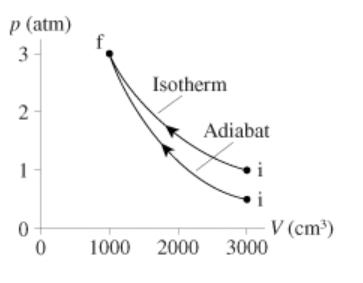# Problem: 0.10 mol of nitrogen gas follow the two processes shown in the figure(Figure 1) .a. How much heat is required for isothermal process?b. How much heat is required for adiabatic process?

###### FREE Expert Solution

Work done:

$\overline{){\mathbf{W}}{\mathbf{=}}{\mathbf{n}}{\mathbf{R}}{\mathbf{t}}{\mathbf{l}}{\mathbf{n}}\mathbf{\left(}\frac{{\mathbf{V}}_{\mathbf{f}}}{{\mathbf{V}}_{\mathbf{i}}}\mathbf{\right)}}$, but

PV = nRT

Therefore,

$\overline{){\mathbf{W}}{\mathbf{=}}{{\mathbf{\left(}}{\mathbf{P}}{\mathbf{V}}{\mathbf{\right)}}}_{{\mathbf{i}}}{\mathbf{l}}{\mathbf{n}}\left(\frac{V}{}\right)}$fVi

95% (144 ratings)###### Problem Details

0.10 mol of nitrogen gas follow the two processes shown in the figure(Figure 1) .a. How much heat is required for isothermal process?

b. How much heat is required for adiabatic process?